### Journal of Genetic Engineering

Current Issue Volume No: 1 Issue No: 1

ISSN: 2694-1198

Review Article Open Access
• Available online freely Peer Reviewed
• ### Migration of a Population

A.N. Volobuev 1

1Samara State Medical University, Department of Medical Physics, Samara, Russia

## Abstract

On the basis of Hardy – Weinberg law the problem of migration from the genetic point of view is considered. It is proved the linear differential equation of migratory process of a panmictic population. The phase of the solution of this equation is investigated. On the basis of the carried out analysis the dependence of migration velocity of a population on average time of alternation of generations is found. It is shown that migration of primitive people from Africa to Europe needed alternation the several hundred generations. The dependence of migration velocity of a population on the average area developed by a population for year is investigated. Lacks of the carried out analysis owing to absence of the account of natural selection and inbreeding are marked.

##### Author Contributions
Received 23 Dec 2019; Accepted 08 Jan 2020; Published 11 Jan 2020;

Academic Editor: Omnia Abd El-Rahman, National Institute of Laser Enhanced Sciences, Cairo University, Egypt

Checked for plagiarism: Yes

Review by: Single-blindThis is an open-access article distributed under the terms of the Creative Commons Attribution License, which permits unrestricted use, distribution, and reproduction in any medium, provided the original author and source are credited.

Competing interests

The authors have declared that no competing interests exist.

Citation:

A.N. Volobuev (2020) Migration of a Population. Journal of Genetic Engineering - 1(1):15-20.

## Introduction

Populations during the vital activity carry out various motions, i.e. migrate in searches of the best life places, at excess of individuals which can exist in the given region, migrate in order to prevent collisions with other populations, for a new territories gain, etc. All history of a human population development is connected to migration of its parts in various regions of Globe.

Migratory processes have the laws and genetic consequences. At migration of populations there is a genes flow 1, 2. The most known example of a migratory processes consequences the change of the human skin pigmentation at migration of people from Africa where their occurrence is supposed in higher latitudes that has been caused by the adaptation of a population to new conditions of existence. At the same time the process of a population motion influenced on its genome.

## Mathematical and Genetic Basis of Population Migration

Not concerning the concrete reasons of migration we shall analyse genic changes in a migrating population under various conditions.

Let's consider the elementary case of migration of the panmictic populations.

Moving of the population individuals is not equivalent to moving of genome. But individuals of the population are carriers of genome therefore moving of the population individuals and moving of genome are closely connected with each other.

Let’s assume that at the migration of the population keeps it panmictic character. Though such assumption is the big idealization but for the initial analysis it is allowable especially if the moving part of the population is great enough i.e. contains a plenty of family trees.

For the solving of our problem it is necessary to have, first of all, the base equation which describes the genome of the moving population. We shall consider requirements which are necessary such equation to satisfy.

First of all, this equation should include Hardy - Weinberg law i.e. to pass in Hardy - Weinberg law at absence of a population movement.

Second, the equation should reflect indifferent character of genome balance for moving panmictic populations.

Thirdly, since movement of a population represents some wave process including elements of individual diffusion in space it is possible to assume that the equation for moving genome should have diffusive-wave character.

For a basis we shall accept the differential equation reflecting balance of genome of the motionless panmictic population or Hardy - Weinberg balance 3: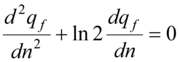…………… (1)

where qf there is frequency of the some recessive allele of two-allele systems inthe Х-chromosome at the woman, n= t/T - dimensionless time, t - current time,Т - the normalizing time equal to the average period of generations alternation in the moving population T  ≈  25 years .

Taking into account required diffusive-wave character of the equation we shall write down the base equation for genome of the moving population as: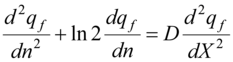…………. (2)

Where D= D*T there is the normalized factor of diffusion, D* - factor of diffusion of the population individuals, and hence of genome in space. This factor is multiplied for normalizing timeТ with the purpose of dimensionless time use in the unit of factor D measurements, X - spatial coordinate of the population moving.

Let's note that the equation (2) simultaneously reflects indifferent character of the genome balance of the moving population since satisfies to the solving qf= const.

The equation (2) allows analyze moving of the population and it genome in the certain direction X that most frequently took place in a history of mankind. If moving of a population occurs simultaneously in different directions it is necessary to use the equation:

……………. (3)

where Δqf there is the Laplacian of the functions qf.

With the purpose of the solving finding of the equation (2) we shall make replacement of the variable under the formula: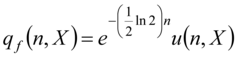……………. (4)

Where u(n,X) there is new variable dependent on dimensionless time and coordinate of moving.

Substituting (4) in (2), we shall receive the equation:……………. (5)

The result of the solution of Cauchy problem for the given equation has rather complex character for the analysis. For example, for initial conditions: at n = 0 the function qf = qf0 = u0 =const, and

(initial constant on time value of recessive allele frequency), and also in spatial area - ∞ < X < ∞ the solution of the equation (5) looks like 4:

……………. (6)

where f there is any function of coordinates x+√Dn and x-√Dn, ξ variable of integration, I1(z)- modified Bessel function of the first order.

Function f(x-√Dn) means a wave of the population genome, and hence the wave of the population spread to the right, and f(x+√Dn) - to the left.

Let’s consider in more detail the form of a migrating population wave. It is obvious that approximation of uniform movement in this case is rather rough. The population goes then after a while stops and develops the certain territory. Then, after an exhaustion of resources the movement renews, etc. On figure 1 the front of a genomic wave of moving population is shown.

Each man is the carrier of all genome. Therefore examined allele frequency we shall assume an identical at each individual of a population qf= qf 0= const. Some individuals, for example, hunters, scouts 1, can leave the basic file of a population 2 on rather big distance. A part of individuals - collectors of a plants fruits 3 leave on smaller distance. In the analysis it is possible to replace such complex character of forward front of a moving population some dotted line shown on figure 1.

Figure 1. Front of a genomic wave of migratory population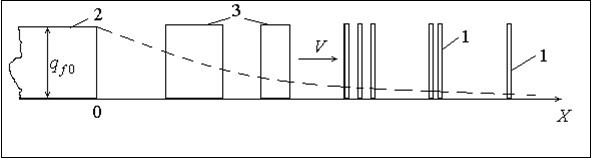For our purposes is allowable to use the exponential kind of function f. Also we shall examine only a wave of the population genome propagating to the right. In this case the solving of the equation (5) can be written down as: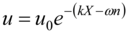…….(7)

In the formula (7) we shall be interested the parameters of the wave phase k and ω instead of the wave front which according to the solution (6) can be anyone. Substituting (7) in (5) we shall find dependence between parameters of the wave phase and factor of diffusionD:……..(8)

Substituting (7) in (4) we shall pass to former function qf:

……….(9)

Once again we shall note that is real any change of function qf is not present since each individual carries a full genome. Function (9) reflects the conditional change shown on figure 1 by dotted line.

In the formula (9) k = 2π/λ there is the wave number and (ω -1/2 ln 2) - dimensionless cyclic frequency of the wave population genome, λ - length of front of the moving population wave. Equality of initial sizes of functions qf0 =u0= const is used also.

First of all we shall find speed of the wave front propagation of population genome. If the population at the movement keeps the panmictic character the Hardy - Weinberg balance is indifferent and should be observed qf=qf0 = const .

Hence, according to (9) the phase of the wave is equal:

………….(10)

Speed of the wave front movement of population genome according to (10), figure 1, is equal:

…………..(11)

Expression (11) has completely general character which has been not connected to the concrete form of a population front wave (9).

Substituting in (11) parameter ω from (8) and taking into account D = D*T we shall find:

…………..(12)

Let's note that in (12) the condition is observed: at D* = 0 the population is motionless V = 0.

Using length of the population wave front under the formula λ = 2π/k we shall find:

…………..(13)

It is interesting to analyse on extremum the function of speed V (T, λ).

Simple transformations of derivatives ∂V/∂T and ∂V/∂λ, and equating of these derivatives with zero result in a trivial conclusion V (T, λ) = 0 that the minimal size of speed is determined by the condition D*= 0 i.e. a motionless population.

On figure 2 the dependence of the speed of the population genome moving on the average period T of the generation alternation in population plotted under formula (13) is shown.

Figure 2. Dependence of the speed of the population genome moving on the average period T of the generation alternation in population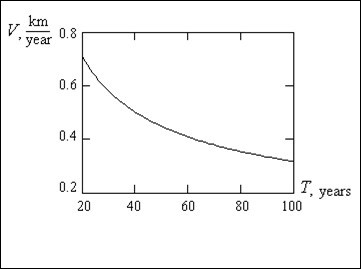At calculation the λ = 0.4km length of the population front wave was accepted factor of diffusion D* = 10Km2/year.

Calculation shows that with increase in the period of a generation alternation the speed of the population and it genome movement to become less. It is correct since younger individuals are more dynamical i.e. if the generations is more often are alternated the population faster move. At the period of the generation alternation T  ≈  25 years the calculated speed of genome movement, and hence the population is equal to V ≈ 0.63 km/year

It is obvious that this speed basically is caused by a gradual exhaustion of resources on a way of the population movement.

It is supposed, that occurrence Homo sapiens has taken place in the central Africa about 300 thousand years ago. About 40 thousand years ago Homo sapiens migrated to Europe. Believing, that during migration from Africa to Europe, figure 3, primitive people have passed about 5000 km it is possible to find the time of movement ~ 8000 years. For this time 320 generations were alternated.

Figure 3. Way of migration of primitive people from Africa to Europe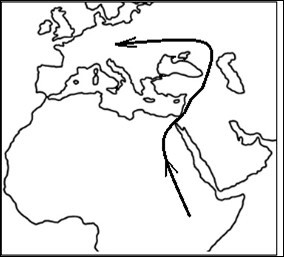On figure 4 the dependence of speed of the population movement on length of the wave front is shown at period of the generation alternation equal T  ≈  25 years, plotted under formula (13). From the graph it is visible that with increase in length of the wave the speed of the population movement falls. It is correct since more compact populations should move with the greater speed than less compact. But this dependence is not so strong.

Figure 4. Dependence of velocity of movement of a population on length of front of a wave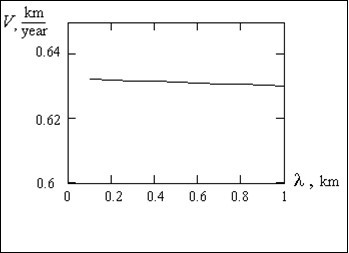At the analysis of the equation (13) there is a question on sense of diffusion factor D*. This parameter reflects the area developed by the population within year at each stage of movement. Actually this parameter reflects the resources necessary for vital activity and movement of the population. We shall notice that the parameter D = D* T is meaning of the area developed by the population during a life of one generation.

On figure 5 dependence of speed of the population movement on diffusion factor D* is shown.

Figure 5. Dependence of speed of a population movement on the area developed by a population within year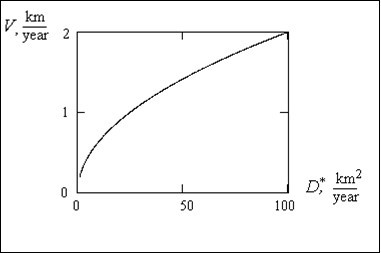The found dependence is rather strong. It shows that the big resource area the population can develop for the year at the movement the faster it move.

## Conclusion

The basic lack of the carried out analysis of the moving population is the assumption about it panmictic character. Actually the migration of the human population part in various directions occurred rather small groups, tribes or separate communities of tribes. At these tribes there was be relative small number of family trees therefore consanguineous mating, i.e. inbreeding has been widespread. But research of process of a population migration with the account of inbreeding and selection 5 has more difficult character. This process concerns to nonlinear genetics.